## Section13.8Driven Oscillator

A damped oscillator will eventually come to rest if no additional energy is supplied to it. To supply energy to the oscillator you could apply any force that will do a net positive work on the oscillator. Among many forces that will do the job, a harmonically time varying force is of particular interest in physics for many applications. A harmonically varying force varies in time sinusoidally with a well-defined frequency, which we will denote by $\omega_d\text{.}$

\begin{equation} F_d = F_0\, \cos(\omega_d\, t).\tag{13.8.1} \end{equation}

where $F_0$ is the amplitude of the force. Note that $\omega_d=0$ corresponds to just a constant force, such as gravity; a constant force will do positive work in one half of the cycle and the same amount of negative work in the second half of the cycle, therefore a constant force will not change the energy of the oscillator over one complete cycle.

Another thing to notice here is that you could think of $(\omega_d t )$ as phase of the driving force. Therefore, we now have the possibilities of oscillator and driving force acting in phase, out of phase, or in-between. These possibilities create interesting effects we will address here. One of those effects is the effect of resonance, which happens when the force is most effective.

### Subsection13.8.1Equation of Motion

Consider a block of mass $m$ attached to a spring of spring constant $k$ subject to a linear drag force and a harmonic driving force. Suppose all the forces act along $x$ axis with origin as the point where the block is in equilibrium. Then, the $x$ component of Newton's second law gives us the following equation of motion.

\begin{equation} m a_x = -k x - b v_x + F_0\,\cos(\omega_d\, t).\label{eq-driven-oscillator-equation-of-motion}\tag{13.8.2} \end{equation}

This equation is even harder to solve for $x(t)$ than was the damped system. We will assume that $m\text{,}$ $k\text{,}$ and $b$ are such that we have underdamped oscillator, i.e., $(b/2m) \lt \sqrt{k/m} \text{.}$ Our purpose usually is to drive this oscillating system and try to put in additional energy so that the motion can continue rather than damp out.

### Subsection13.8.2Solution of the Equation of Motion

The solution of Eq. (13.8.2) has two parts, a complimentary solution, to be denoted by $x_c$ and a particular solution, to be denoted by $x_p\text{.}$

\begin{equation} x = x_c + x_p.\tag{13.8.3} \end{equation}

The complimentary part is the solution of $x$ in the absence of the driving force, which we have already studied in the underdamping oscillator.

\begin{equation} x_c = A e^{-\gamma t /2}\, \cos(\omega^{\prime} t + \phi ),\tag{13.8.4} \end{equation}

where

\begin{equation*} \omega = \sqrt{\dfrac{k}{m}},\ \ \gamma = \dfrac{b}{m},\ \ \omega^{\prime} = \sqrt{ \omega^2 - \gamma^2/4 }, \end{equation*}

and $A$ and $\phi$ depend on the initial conditions, $x_0$ and $v_0\text{.}$

The particular solution $x_p$ takes into account the driving force and oscillates at the frequency of the force but may or may not be in phase with the force. This solution looks more like a free oscillator solution. We can write this solution either as (1) amplitude/phase form or (2) two-amplitude form.

\begin{align} \amp \text{(1) }\ \ x_p = X_d\, \cos( \omega_d t + \phi_d), \label{eq-driven-oscillator-eom-solution-amp-ph}\tag{13.8.5}\\ \amp \text{(2) }\ \ x_p = A_{\text{in}}\, \cos( \omega_d t) + A_{\text{out}}\, \sin( \omega_d t),\label{eq-driven-oscillator-eom-solution-two-amps}\tag{13.8.6} \end{align}

where the two-amplitude-form has an in-phase amplitude $A_{\text{in}}$ and an out-of-phase amplitude $A_{\text{out}} \text{,}$ which refer to the parts that swing in sync with the driving force and that are $\pi/2$ radian, i.e., a quarter cycle off. There is a lot of work involved in obtaining the formulas for the four constants. We will just quote the answer here.

We will write the expressions for $X_d\text{,}$ $\phi_d \text{,}$ $A_\text{in}\text{,}$ and $A_\text{out} \text{,}$ using the following quantity, $D\text{,}$ which stands for “denominator”.

\begin{equation} D = \left( \omega^2 - \omega_d^2 \right)^2 + \left( \gamma \omega_d\right)^2.\tag{13.8.7} \end{equation}

So, here are the expressions:

\begin{align} \amp X_d = \dfrac{F_0/m}{ D},\ \ \ \tan \phi_d = -\dfrac{\gamma \omega_d}{\omega^2 - \omega_d^2},\ \ \ \tag{13.8.8}\\ \amp A_{\text{in}} = \dfrac{F_0}{m}\ \dfrac{\left(\omega^2 - \omega_d^2\right)}{ D},\ \ \ A_{\text{out}} = \dfrac{F_0}{m}\ \dfrac{\gamma \omega_d}{ D}.\label{eq-driven-oscillator-eom-solution-two-amps-wd-formulas}\tag{13.8.9} \end{align}

### Subsection13.8.3Long Term Steady Behavior and Short Term Transients

Since $x_c$ is simply the damped oscillator and $x_p$ is perpetually oscillating, $x_c$ will become negligily small in time compared to $x_p\text{.}$ Therefore, for sufficiently large times, we can just drop $x_c$ and assume $x \approx x_p\text{.}$ We call this condition the steady state condition , and call $x_p$ the steady state solution.

\begin{equation} x(t) = x_c + x_p\ \ \ \overset{\text{after sufficiently long time}}{\rightarrow\rightarrow\rightarrow\rightarrow\rightarrow\rightarrow\rightarrow\rightarrow\rightarrow\rightarrow} \ \ \ x_p.\tag{13.8.10} \end{equation}

How long do we have to wait till $x_c$ part is no longer needed? The analysis of solution shows that when $t \gt\gt 1/\gamma \text{,}$ you can drop $x_c \text{.}$ The remaining part $x_p$ given in Eq. (13.8.5) or in Eq. (13.8.6) is just an oscillator at the frequency of the driving force - the driver finally succeeds in forcing the block to oscillate at the driving frequency as illustrated in Figure 13.8.1.

Before $t$ is long enough that justifies dropping $x_c\text{,}$ the displacment of the oscillator, $x(t) \text{,}$ has significant influence from the initial conditions $x_0$ and $v_0\text{.}$ Usually, the motion of the block is not even a simple harmonic motion. These initial patterns are called transients since, they will die out with time and eventually, we would get the steady harmonic oscillation, as illustrated in Figure 13.8.1.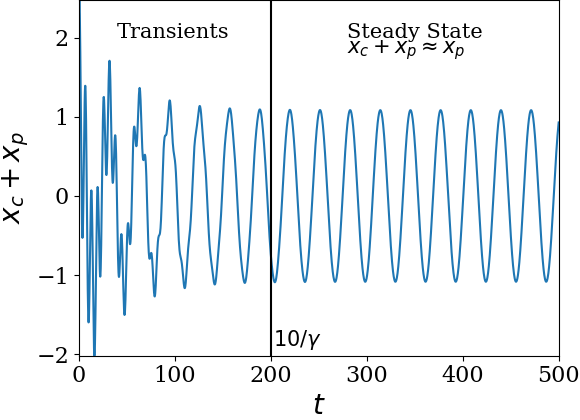Figure 13.8.1. A plot of position versus time for a driven underdamoed oscillator with parameters $m=1\text{,}$ $k=1\text{,}$ $b = 0.05\text{,}$ $F_0 = 1\text{,}$ and initial conditions, $x_0 = 1$ and $v_0 = 1\text{.}$ The initial transients give way to the steady behavior beyond $t \gt\gt 1/\gamma\text{,}$ where $\gamma = b/m\text{.}$ The vertical line at $t = \dfrac{10}{\gamma}$ shows that $t \gt 10/\gamma$ is sufficient for steady state condition.

### Subsection13.8.4Steady State and Resonance of Amplitude

From Eq.(13.8.5), we can tell that, in the steady state, the block oscillates at the driving angular frequency $\omega_d$ between $x=-X_d$ and $x=X_d$ with

\begin{equation} X_d = \dfrac{F_0/m}{ \left( \omega^2 - \omega_d^2 \right)^2 + \left( \gamma \omega_d\right)^2 }.\label{eq-steady-state-amplitude-as-func-omega}\tag{13.8.11} \end{equation}

Clearly, the amplitude of block's steady state oscillations depend on the strength of the force through $F_0/m\text{.}$ But, what is interesting is that it also depends on the driving frequency $\omega_d\text{.}$ That is, you can get different amplitudes of oscillation for the same magnitude of the force $F_0$ if you apply the force at a different frequency. You can definitely tune the driving frequency so that you get the maximum impact.

Figure 13.8.2 shows the amplitude $X_d$ versus the driving frequency $\omega_d\text{.}$ I have plotted frequency in units in which $\omega = \sqrt{k/m} = 1\text{.}$ Clearly, there is a frequecy at which $x_d$ has the maximum value. This is where the energy of the oscillator will be maximum, i.e., $\dfrac{1}{2}k X_{\text{d,max}}^2\text{.}$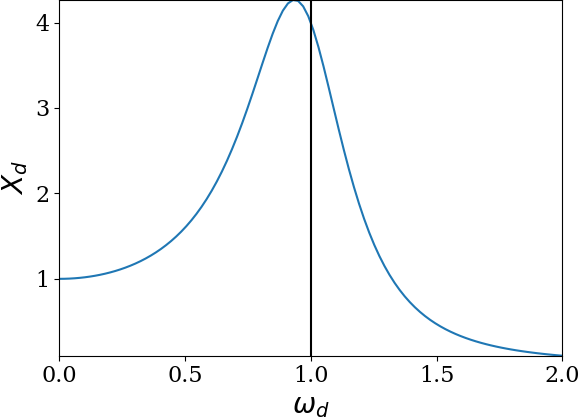Figure 13.8.2. A plot of the steady state amplitude $X_d$ versus the driving frequancy $\omega_d\text{.}$ The angular frequency is in units of $\omega = \sqrt{k/m}\text{.}$ That is $\omega_d = 1$ refers to $\omega_d = \omega\text{.}$ The oscillators have $m=1\text{,}$ $k=1\text{,}$ $b = 0.5\text{.}$ The driving force has amplitude $F_0 = 1$ and $\omega_d$ is varied. The displacement $x$ of the block is observed after $t \gt\gt 1/\gamma\text{,}$ where $\gamma = b/m\text{.}$

At what frequency does this happen? Take a look at Eq. (13.8.11), this will happen at $\omega_d$ at which the dnominator will be minimum. This happens at the following value of $\omega_d\text{,}$ which we call the resonance frequency of the amplitude. We will denote this value of $\omega_d$ by $\left( \omega_R \right)_{X_d} \text{.}$

\begin{equation} \left( \omega_R \right)_{X_d} = \sqrt{ \omega^2 - \gamma^2 }.\tag{13.8.12} \end{equation}

There are other resonance frequencies for other properties, such as power, which occur at different values of $\omega_d$. Perhaps a more important resonance is the resonance of the average power of the driving force. This tells us the frequency at which, on average, energy can be delivered at the highest rate.

### Subsection13.8.5Resonance of Average Power

Average power is obtained by averaging instantaneous power over one cycle in the steady state. The instantaneous power of the driving force is

\begin{equation} P(t) = F_d\, v_{p,x},\tag{13.8.13} \end{equation}

where $v_{p,x}$ is the steady state velocity of the block. It is better to get the steady state velocity $v_x$ from the two-amplitude form of $x_p$ in Eq. (13.8.6) since here thinking in terms of in-phase and out-of-phase is very helpful. The velocity (obtained by derivative if you know calculus otherwise we just accept this as another formula) is

\begin{equation} v_{p,x} = \omega_d\, A_{\text{out}}\, \cos( \omega_d t) -\omega_d\,A_{\text{in}}\, \sin( \omega_d t).\tag{13.8.14} \end{equation}

Now, upon multiplication with $F_d = F_0\, \cos(\omega_d t)\text{,}$ we get

\begin{align} P(t) = \omega_d\,\amp F_0\, A_{\text{out}}\, \cos^2( \omega_d t) \notag\\ \amp - \omega_d\,F_0\,A_{\text{in}}\, \cos( \omega_d t)\sin( \omega_d t).\tag{13.8.15} \end{align}

The second term on the right is actually one sine with twice the frequency. Hence, it averages out to zero. the first term, upon averaging gives the average power.

\begin{equation} P_{\text{av}} = \dfrac{1}{2}\omega_d\,F_0\, A_{\text{out}}.\tag{13.8.16} \end{equation}

Now, using the expression of $A_{\text{out}}$ from Eq. (13.8.9), we have

\begin{equation} P_{\text{av}} = \dfrac{1}{2m}\, \dfrac{\gamma\, \omega_d^2\, F_0^2}{\left( \omega^2 - \omega_d^2 \right)^2 + \left( \gamma \omega_d\right)^2 } .\tag{13.8.17} \end{equation}

A plot of average power versus the driving frequency is shown in Figure 13.8.3. The resonance of power occurs at $\omega_d = \omega\text{.}$ This frequency, in this context, is called the resonance frequency of power. We will denote this by $\left( \omega_R\right)_{P_{\text{av}}} \text{.}$ One can prove analytically that average power $P_{\text{av}}$ as a function of $\omega_d$ has maximum at

\begin{equation} \left( \omega_R\right)_{P_{\text{av}}} = \omega = \sqrt{\dfrac{k}{m}}.\tag{13.8.18} \end{equation}

It is important to note that the natural frequency of the oscillator $\sqrt{k/m}$ is also the resonance frequency of average power. Many systems take this fact into account. Some examples are: tuning a piano, Laser, Magnetic Resonance Imaging, etc.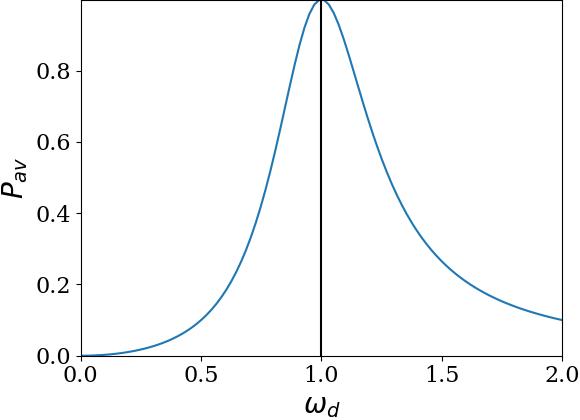Figure 13.8.3. A plot of the average power $P_{text{av}}$ of the driving force in steady state versus the driving frequancy $\omega_d\text{.}$ The angular frequency is in units of $\omega = \sqrt{k/m}\text{.}$ That is $\omega_d = 1$ refers to $\omega_d = \omega\text{.}$ The resonance occurs at $\omega_d = \omega\text{.}$ The oscillators have $m=1\text{,}$ $k=1\text{,}$ $b = 0.5\text{.}$ The driving force has amplitude $F_0 = 1$ and $\omega_d$ is varied. The displacement $x$ of the block is observed after $t \gt\gt 1/\gamma\text{,}$ where $\gamma = b/m\text{.}$

The resonance curve of average power for three different values of the damping parameter $\gamma\text{.}$ Lower values of $\gamma$ refers to lower level of damping. As shown in Figure 13.8.4, the resonance curve becomes steeper with less damping.

By solving $P_\text{av} = \frac{1}{2}P_{\text{av, max}}\text{,}$ we find the width of power resonance curve at half max, called full-width at half maximmum (FWHM).

\begin{equation*} P_\text{av} = \dfrac{1}{2}P_{text{av,max}}, \end{equation*}

with

\begin{equation*} P_\text{av,max} = \dfrac{F_0^2}{2 m \gamma}. \end{equation*}

Solving for $\omega_d\text{,}$ we get two values of $\omega_d\text{,}$ one on each side of the resonance frequency, whose range gives the width.

\begin{equation*} \text{FWHM} = \gamma. \end{equation*}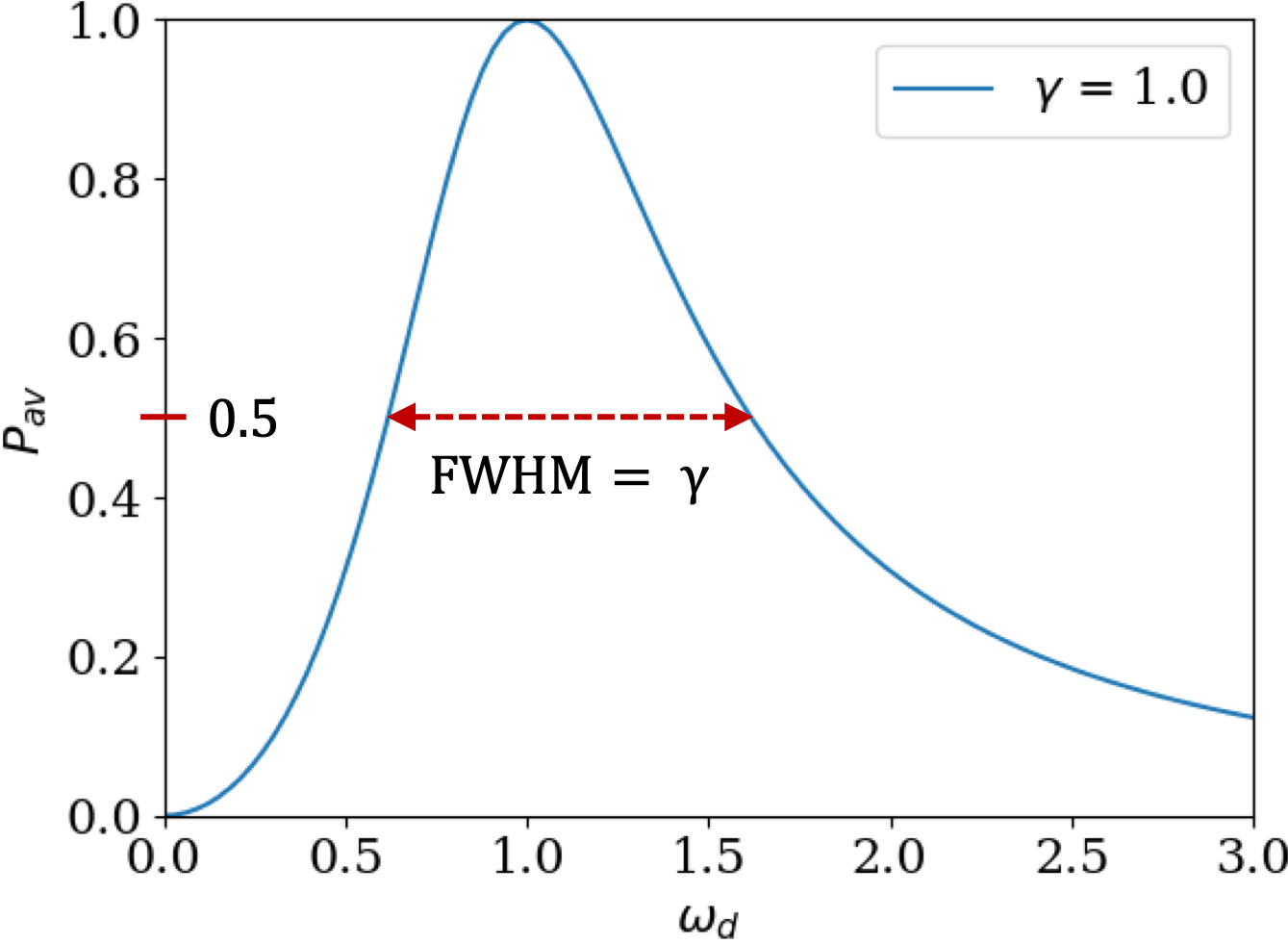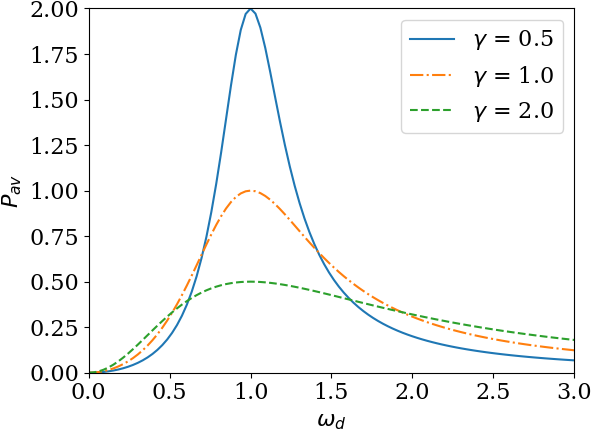Figure 13.8.4. A plot of the average power $P_{text{av}}$ of the driving force at different values of the damping parameter $\gamma\text{.}$ With lowering of damping, the curve becomes steeper and rises higher. Other parameters were kept same for all three: $F_0/2m = 1$ and $\omega=1\text{.}$ The width of power resunance curve at half of maximum power in each case is equal to the damping parameter $\gamma\text{.}$

A block of mass $0.2\text{ kg}$ is hung from a spring of spring constant $100\text{ N/m}\text{.}$ The block is subject to a viscous drag, $F_{\text{visc}} = - b v\text{,}$ with $b = 3\text{ N.s/m}\text{.}$ A driving force $F_d = F_0\,\cos(\omega_d t)\text{,}$ with $F_0 = 5\text{ N}$ and $\omega_d = 20\text{ rad/s}\text{,}$ also acts on the block.

(a) What is the amplitude of oscillations of the block in the steady state?

(b) If frequency $\omega_d$ is varied, at what value of $\omega_d$ is the steady state amplitude largest?

(c) If frequency $\omega_d$ is varied, at what value of $\omega_d$ average power delivered by the driving force is the largest?

Hint

(a) Use formula for $X_d\text{.}$ (b) Use resonance of amplitude. (c) Use resonance of average power.

(a) $0.08\text{ m}\text{,}$ (b) $16.6\text{ sec}^{-1} \text{,}$ (c) $22.36\text{ sec}^{-1}\text{.}$

Solution 1 (a)

(a) We just plug in the numbers in the formula for $X_d\text{.}$ But first we find the values of the effective parameters.

\begin{align*} \omega \amp = \sqrt{ \dfrac{k}{m} } = \sqrt{ \dfrac{100}{0.2} } = 22.36\text{ sec}^{-1},\\ \gamma \amp = \dfrac{b}{m} = \dfrac{3}{0.2} =15\text{ sec}^{-1}, \end{align*}

Using these in the formulas for $X_d$ we get

\begin{equation*} X_d = \dfrac{5/0.2}{\sqrt{ ( 22.36^2 - 20^2 )^2 + ( 20\times 15 )^2 }} = 0.08\text{ m}. \end{equation*}
Solution 2 (b)

(b) We seek the value of $\omega_{R,X_d} \text{.}$

\begin{equation*} \omega_{R,X_d} = \sqrt{ \omega^2 - \gamma^2 } = \sqrt{ 22.36^2 - 15^2 }=16.6\text{ sec}^{-1}. \end{equation*}
Solution 3 (c)

(c) The resonance of average power occurs at angular frequency $\omega = 22.36\text{ sec}^{-1}\text{.}$

A harmonically driven lightly damped oscillator has a resonance frequency of average power at $100\text{ Hz}\text{.}$ The width of the power curve is $\pi\text{ s}^{-1}\text{.}$ Find the quality of the oscillator.

Hint

Use properties of the power resonance curve.

$200\text{.}$
Since width of power resonance curve is equal to the damping parameter $\gamma\text{.}$